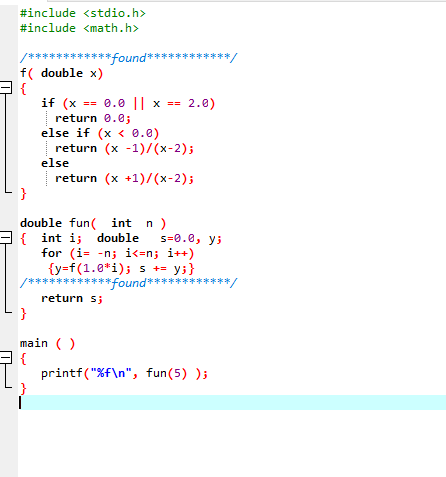mycode.c: 在函数‘main’中:
mycode.c:14: 警告：格式‘%f’需要类型‘float *’，但实参 2 的类型为‘double’
mycode.c:16: 错误：expected expression before ‘int’
mycode.c:16: 警告：语句不起作用
mycode.c:17: 错误：expected ‘;’ before ‘{’ token

#include <stdio.h>
int main()
{
float score;
pirntf("请输入学生成绩");
scanf("%f",&score);
while(score>100||score<0)
{printf("输入数据有误");
scanf("%f",score);
}
switch(int)(score/10)
{case 10:
case 5:
case 4:
case 3:
case 2:
case 1:
}

//int x  =0;
//int y = 0;
//scanf("%d", &x);
//结果输出使用prinf
//printf("%d",x);
return 0;
}

09/02/2019

#include <stdio.h>
#include<string.h>
#define N 10
char str[N];
int main()
{void sort(char[]);
int i,flag;
for(flag=1;flag==1;)
{
scanf("%s",&str);
if(strlen(str)<=N)
flag=0;
}
sort(str);
for(i=0;i<N;i++)
printf("%c",str[i]);

return 0;
}
void sort(char str[])
{
int i,j;
char t;
for(j=1;j<N;j++)
for(i=0;(i<N-j)&&(str[i]!='\0');i++)
if(str[i]>str[i+1])
{
t=str[i];
str[i]=str[i+1];
str[i+1]=t;

}
}05/26/2019

#include <stdio.h>
int main()
{
int k1,k2,k3,k4,k5,k6,k7,k8,k9,k10;
int i,a,n,s;
for (a=2;a<=1000;a++)
{n=0;
s=a;
for (i=1;i<a;i++)
if (a%i==0)
{n++;
s=s-i;
switch(n)
{case 1:
k1=i;  break;
case 2:
k2=i;  break;
case 3:
k3=i;  break;

case 4:
k4=i;  break;
case 5:
k5=i;  break;
case 6:
k6=i;  break;
case 7:
k7=i;  break;
case 8:
k8=i;  break;
case 9:
k9=i;  break;
case 10:
k10=i;  break;
}
}
if (s==0)
{
printf("%d its factors are ",a);
if (n>1)  printf("%d,%d",k1,k2);
if (n>2)  printf(",%d",k3);
if (n>3)  printf(",%d",k4);
if (n>4)  printf(",%d",k5);
if (n>5)  printf(",%d",k6);
if (n>6)  printf(",%d",k7);
if (n>7)  printf(",%d",k8);
if (n>8)  printf(",%d",k9);
if (n>9)  printf(",%d",k10);
printf("\n");
}
}
return 0;
}

……处有未经处理的异常…… 写入位置……03/28/2018

#include<stdio.h>
int main()
{
int x,y,q,z,e;

while(scanf("%d %d",&x,&y) == 2){
if (x!=0||y!=0)
printf("%d",z=x*y);
else break;
}
{
while(e!=0){
e=x%y;
x=y;
y=e;
}

q=z/x;
printf("%d",q);
return 0;
}}09/08/2017

*

***

*****

***

*

05/08/201704/21/2017

#include<stdio.h>

int main()
{
int a,b,c,num,n;
printf("请输入一个三位数:\n");
scanf("%d",&num);
c=num%10;
b=(num/10)%10;
a=num/100;
if(a==b&&a!=c)
printf("n=1");
else if(a==c&&a!=b)
printf("n=1");
else if(b==c&&a!=b)
printf("n=1");
else
printf("n=0");
return 0;
}

03/10/201703/07/2017

LINK : fatal error LNK1104: cannot open file "Debug/Guess.exe"

Guess.exe - 1 error(s), 0 warning(s)

02/18/2017

## 欢迎大家交流方法！！

06/09/2014

### 推荐贴吧

#### 技术动态吧

• Trustie(确实)
• QQ群：1071514693